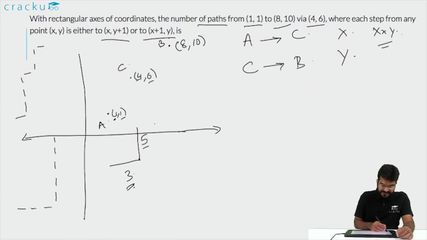Question 97

# With rectangular axes of coordinates, the number of paths from (1, 1) to (8, 10) via (4, 6), where each step from any point (x, y) is either to (x, y+1) or to (x+1, y), is

Solution

The number of paths from (1, 1) to (8, 10) via (4, 6) = The number of paths from (1,1) to (4,6) * The number of paths from (4,6) to (8,10)

To calculate the number of paths from (1,1) to (4,6), 4-1 =3 steps in x-directions and 6-1=5 steps in y direction

Hence the number of paths from (1,1) to (4,6) = $$^{(3+5)}C_3$$ = 56

To calculate the number of paths from (4,6) to (8,10), 8-4 =4 steps in x-directions and 10-6=4 steps in y direction

Hence the number of paths from (4,6) to (8,10) = $$^{(4+4)}C_4$$ = 70

The number of paths from (1, 1) to (8, 10) via (4, 6) = 56*70=3920

### View Video Solution• All Quant CAT Formulas and shortcuts PDF
• 30+ CAT previous papers with solutions PDF